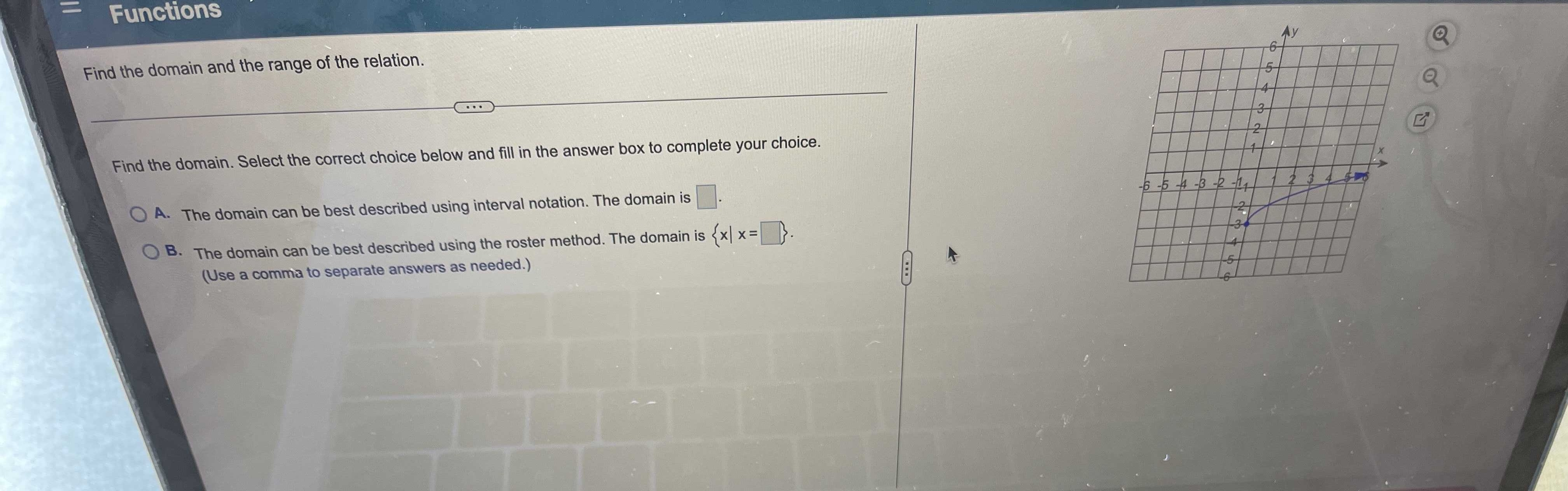### Still have math questions?

Algebra
QuestionFind the domain and the range of the relation.

A. The domain can be best described using interval notation. The domain is ______.

B. The domain can be best described using the roster method. The domain is {x|x=_____}. (Use a comma to separate answers as needed Belect.)

A. $$[0,\infty )$$